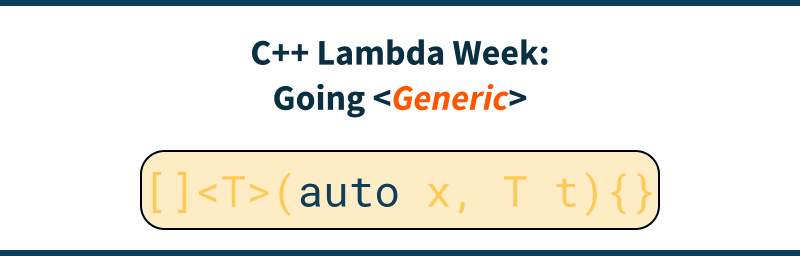# C++ Lambda Week: Going Generic

See my new website at cppstories.comWe’re in the third day of the lambda week. So far, you’ve learned basic syntax and how to capture things. Another important aspect is that lambdas can also be used in the “generic” scenarios. This is especially possible since C++14 where we got generic lambdas (`auto` arguments), and then in C++20, you can even specify a template lambda!

## The Series

This blog post is a part of the series on lambdas:

## Auto Return Type Deduction

The first crucial part of lambdas that allows you to use them in a “generic” context is the return type deduction.

Even since C++11 (although in a simplified form initially) you could write:

``auto lam = [](int x) { return x * 1.1; }``

And don’t bother with the return type. The compiler can deduce `double` in the above case.

In C++14, we even got `auto` return type for all functions, so they share the common logic with lambdas.

Such a feature is necessary when you want to call your lambda in templated code when specifying the return type might be tricky.

## Generic Lambdas in C++14

The early specification of Lambdas allowed us to create anonymous functional objects and pass them to various generic algorithms from the Standard Library. However, closures were not “generic” on their own. For example, you couldn’t specify a template parameter as a lambda parameter.

Fortunately, since C++14, the Standard introduced Generic Lambdas and now we can write:

``````const auto foo = [](auto x) { std::cout << x << '\n'; };
foo(10);
foo(10.1234);
foo("hello world");``````

Please notice `auto x` as a parameter to the lambda. This is equivalent to using a template declaration in the call operator of the closure type:

``````struct {
template<typename T>
void operator()(T x) const {
std::cout << x << '\n';
}
} someInstance;``````

If there are more `auto` arguments, then the code expands to separate template parameters:

``const auto fooDouble = [](auto x, auto y) { /*...*/ };``

Expands into:

``````struct {
template<typename T, typename U>
void operator()(T x, U y) const { /*...*/ }
} someOtherInstance;``````

## Template Lambdas

With C++14 and generic lambdas, there was no way to change the `auto` template parameter and use “real” template arguments. With C++20 it’s possible:

For example, how can we restrict our lambda to work only with vectors of some type?

We can write a generic lambda:

``````auto foo = [](auto& vec) {
std::cout<< std::size(vec) << '\n';
std::cout<< vec.capacity() << '\n';
};``````

But if you call it with an `int` parameter (like `foo(10);`) then you might get some hard-to-read error:

``````prog.cc: In instantiation of
'main()::<lambda(const auto:1&)> [with auto:1 = int]':
prog.cc:16:11:   required from here
prog.cc:11:30: error: no matching function for call to 'size(const int&)'
11 | std::cout<< std::size(vec) << '\n';``````

In C++20 we can write:

``````auto foo = []<typename T>(std::vector<T> const& vec) {  // <T> syntax!
std::cout<< std::size(vec) << '\n';
std::cout<< vec.capacity() << '\n';
};``````

The above lambda resolves to a templated call operator:

``````<typename T>
void operator()(std::vector<T> const& s) { ... }``````

The template parameter comes after the capture clause `[]`.

If you call it with `int` (`foo(10);`) then you get a nicer message:

``note:   mismatched types 'const std::vector<T>' and 'int'``

Another important aspect is that in the generic lambda example, you only have a variable and not its template type. If you want to access the type, you have to use `decltype(x)` (for a lambda with `(auto x)` argument). This makes code more wordy and complicated.

For example:

``````// C++17
auto ForwardToTestFunc = [](auto&& ...args) {
// what's the type of `args` ?
return TestFunc(std::forward<decltype(args)>(args)...);
};``````

but with template lambdas there’s not need for that:

``````// C++20:
auto ForwardToTestFunc = []<typename ...T>(T&& ...args) {
return TestFunc(std::forward<T>(args)...); // we have all the types!
};``````

As you can see, template lambdas provide cleaner syntax and better access to types of arguments.

Since Lambdas got very similar syntax to regular functions, at least for the argument part, it’s also possible to use concepts! For example in the terse syntax with constrained `auto`:

``auto GenLambda = [](std::signed_integral auto param) { return param * param + 1; };``

## Summary

In this blog post we revised three elements that make Lambdas generic:
• return type deduction
• generic lambdas - auto function arguments
• template lambdas since C++20
But, I skipped one aspect... do you know what can it be? How about captures? are they generic? I'll leave that question for you :)

And some more things to consider:

Do you use lambdas in a generic context?

Have you tried template lambdas?

Share your experience in comments below the article.

## Next Time

In the next article, you’ll see some tricks with lambdas. See here: Some tricks.

## See More in Lambda Story

If you like to know more, you can see my book on Lambdas! Here are the options on how to get it and join 1000 of readers:

If you want to get additional C++ resources, exlusive articles, early access content, private Discord server and weekly curated news, check out my Patreon website: (see all benefits):